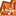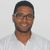# App Development

Announcements
Now Live: Qlik Sense SaaS Simplified Authoring – Analytics Creation for Everyone: READ DETAILS
cancel
Showing results for
Did you mean:Creator

## Quarter Calculations

Hi all,

As many other people, I am also new in the app development with Qlik Sense and I am currently experiencing an issue, concerning quarters and some calculations based on them.

My data is such that it is not possible to create a calendar in Qlik Sense and I need to use the Quarter field that I already have in my excel table.

The Quarter field is in the format - '2013Q1' , '2013Q2', '2013Q3','2013Q4', '2014Q1', etc...

I have altered the script a bit in order to create another field (NumericQuarterField), based on the initial Quarter field that I have and I have removed the "Q" so that it can be a numeric field, so on the currently field I have this format - '20131' , '20132', '20133','20134', '20141', etc...

I have used that numeric field to do a couple of calculations, for example Year over Year growth:

(sum({<NumericQuarterField={\$(=max(NumericQuarterField))}>} Data) - sum({<NumericQuarterField={\$(=max(NumericQuarterField)-10)}>} Data))/ sum({<NumericQuarterField={\$(=max(NumericQuarterField)-10)}>} Data)

The calculation works pretty fine and shows correct results, but then the issue comes when I add a filter, based on the Quarter field (the one that has 'Q' in the name of the Quarter) because if you make a selection in the filter pane, for instance - "2013Q2", Qlik Sense doesn't actually recognize that this is "20132" and shows null values.

As I am pretty sure that the issue comes from the 'max' function I have added in order for the calculation to be performed, do you have any other suggestions on how I can alter the calculation expression so that it can work with the filter pane based on the Quarter field?

Labels (3)

• ### quarter

1 Solution

Accepted SolutionsPartner - Contributor III

Try ...
(sum({<NumericQuarterField={\$(=max(NumericQuarterField))},Quarter=>} Data)

-

sum({<NumericQuarterField={\$(=max(NumericQuarterField)-10)},Quarter=>} Data))

/ sum({<NumericQuarterField={\$(=max(NumericQuarterField)-10)},Quarter=>} Data)

Regards,

GAbriel

3 RepliesMVP

can you share a sample data?

Regards,
Taoufiq ZARRA

"Please LIKE posts and "Accept as Solution" if the provided solution is helpful "

(you can mark up to 3 "solutions") 😉Partner - Contributor III

Try ...
(sum({<NumericQuarterField={\$(=max(NumericQuarterField))},Quarter=>} Data)

-

sum({<NumericQuarterField={\$(=max(NumericQuarterField)-10)},Quarter=>} Data))

/ sum({<NumericQuarterField={\$(=max(NumericQuarterField)-10)},Quarter=>} Data)

Regards,

GAbrielCreator
Author

Hello Gabriel,

Perfect! Thank you very much, it worked flawlessly!

Have a wonderful day!Tags